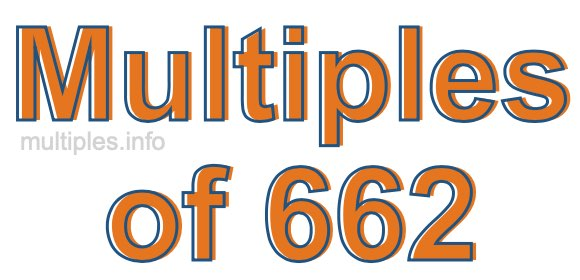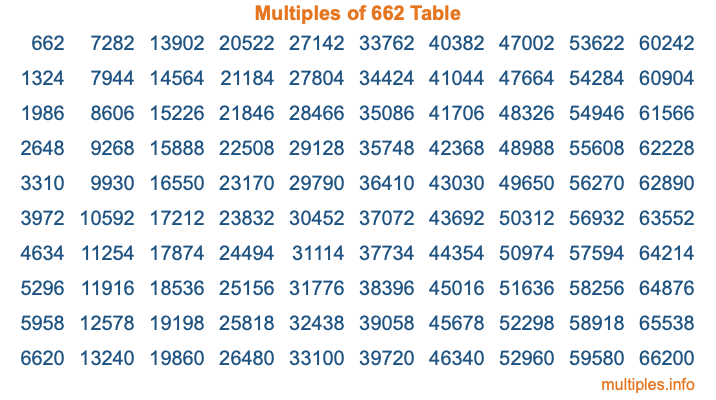Multiples of 662Welcome to the Multiples of 662 page. Here we will first teach you everything you will ever need to know about the multiples of 662, and then give you a study guide summary of everything we taught you to make sure you remember it all. Use this page to look up facts and learn information about the multiples of 662. This page will make you a multiples of six hundred sixty-two expert!

Definition of Multiples of 662
Multiples of 662 are all the numbers that when divided by 662 equal an integer. Each of the multiples of 662 are called a multiple. A multiple of 662 is created by multiplying 662 by an integer.

Therefore, to create a list of multiples of 662, you start with 1 multiplied by 662, then 2 multiplied by 662, then 3 multiplied by 662, and so on for as long as you want. Thus, the list of the first five multiples of 662 is 662, 1324, 1986, 2648, and 3310. To see a larger list of multiples of 662, see the printable image of Multiples of 662 further down on this page. We also have a category where you can choose any nth multiple of 662.

Multiples of 662 Checker
The Multiples of 662 Checker below checks to see if any number of your choice is a multiple of 662. In other words, it checks to see if there is any number (integer) that when multiplied by 662 will equal your number. To do that, we divide your number by 662. If the the quotient is an integer, then your number is a multiple of 662.

Is  a multiple of 662?

Least Common Multiple of 662 and ...
A Least Common Multiple (LCM) is the lowest multiple that two or more numbers have in common. This is also called the smallest common multiple or lowest common multiple and is useful to know when you are adding our subtracting fractions. Enter one or more numbers below (662 is already entered) to find the LCM.

Check out our LCM Calculator if you need more details about the Least Common Multiple or if you need the LCM for different numbers for adding and subtraction fractions.

nth Multiple of 662
As we stated above, 662 is the first multiple of 662, 1324 is the second multiple of 662, 1986 is the third multiple of 662, and so on. Enter a number below to find the nth multiple of 662.

th multiple of 662

Multiples of 662 vs Factors of 662
662 is a multiple of 662 and a factor of 662, but that is where the similarities end. All postive multiples of 662 are 662 or greater than 662. All positive factors of 662 are 662 or less than 662.

Below is the beginning list of multiples of 662 and the factors of 662 so you can compare:

Multiples of 662: 662, 1324, 1986, 2648, 3310, etc.

Factors of 662: 1, 2, 331, 662

As you can see, the multiples of 662 are all the numbers that you can divide by 662 to get a whole number. The factors of 662, on the other hand, are all the whole numbers that you can multiply by another whole number to get 662.

It's also interesting to note that if a number (x) is a factor of 662, then 662 will also be a multiple of that number (x).

Multiples of 662 vs Divisors of 662
The divisors of 662 are all the integers that 662 can be divided by evenly. Below is a list of the divisors of 662.

Divisors of 662: 1, 2, 331, 662

The interesting thing to note here is that if you take any multiple of 662 and divide it by a divisor of 662, you will see that the quotient is an integer.

Multiples of 662 Table
Below is an image of the first 100 multiples of 662 in a table. The table is in chronological order, column by column. The first column has the first ten multiples of 662, the second column has the next ten multiples of 662, and so on.The Multiples of 662 Table is also referred to as the 662 Times Table or Times Table of 662. You are welcome to print out our table for your studies.

Negative Multiples of 662
Although not often discussed or needed in math, it is worth mentioning that you can make a list of negative multiples of 662 by multiplying 662 by -1, then by -2, then by -3, and so on, to get the following list of negative multiples of 662:

-662, -1324, -1986, -2648, -3310, etc.

Multiples of 662 Summary
Below is a summary of important Multiples of 662 facts that we have discussed on this page. To retain the knowledge on this page, we recommend that you read through the summary and explain to yourself or a study partner why they hold true.

There are an infinite number of multiples of 662.

A multiple of 662 divided by 662 will equal a whole number.

662 divided by a factor of 662 equals a divisor of 662.

The nth multiple of 662 is n times 662.

The largest factor of 662 is equal to the first positive multiple of 662.

662 is a multiple of every factor of 662.

662 is a multiple of 662.

A multiple of 662 divided by a divisor of 662 equals an integer.

662 divided by a divisor of 662 equals a factor of 662.

Any integer times 662 will equal a multiple of 662.

Multiples of a Number
Here you can get the multiples of another number, all with the same attention to detail as we did for multiples of 662 on this page.

Multiples of
Multiples of 663
Did you find our page about multiples of six hundred sixty-two educational? Do you want more knowledge? Check out the multiples of the next number on our list!

Copyright  |   Privacy Policy  |   Disclaimer  |   Contact